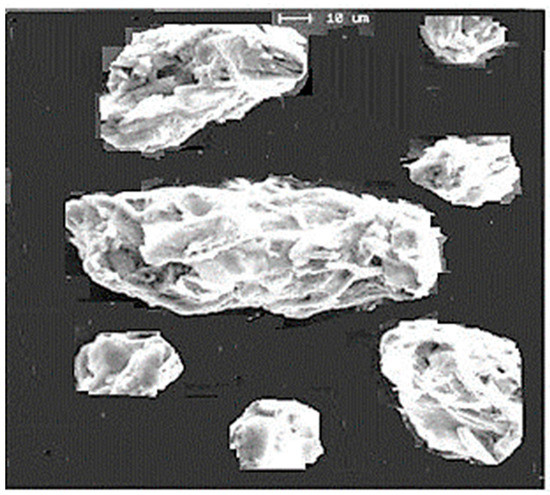Previous Article in Journal
Acknowledgement to Reviewers of Fractal Fract in 2018
Open AccessArticle

Study of Fractal Dimensions of Microcrystalline Cellulose Obtained by the Spray-Drying Method

Celdesigner Ltd, 2 Bergman St., Rehovot 7670504, Israel
Fractal Fract 2019, 3(1), 3; https://doi.org/10.3390/fractalfract3010003
Received: 8 December 2018 / Revised: 11 January 2019 / Accepted: 22 January 2019 / Published: 24 January 2019
In this research, the fractal structure of beads of different sizes obtained by the spray-drying of aqueous dispersions of microcrystalline cellulose (MCC) was studied. These beads were formed as a result of the aggregation of rod-shaped cellulose nanocrystalline particles (CNP). It was found that increasing the average radius (R) of the formed MCC beads resulted in increased specific pore volume (P) and reduced apparent density (ρ). The dependences of P and ρ on the scale factor (R/r) can be expressed by power-law equations: P = Po (R/r)E−Dp and ρ = d (R/r)Dd−E, where the fractal dimensions Dp = 2.887 and Dd = 2.986 are close to the Euclidean dimension E = 3 for three-dimensional space; r = 3 nm is the radius of the cellulose nanocrystalline particles, Po = 0.03 cm3/g is the specific pore volume, and d = 1.585 g/cm3 is the true density (specific gravity) of the CNP, respectively. With the increase in the size of the formed MCC beads, the order in the packing of the beads was distorted, conforming to theory of the diffusion-limited aggregation process. View Full-Text
Show FiguresFigure 1

MDPI and ACS Style

Ioelovich, M. Study of Fractal Dimensions of Microcrystalline Cellulose Obtained by the Spray-Drying Method. Fractal Fract 2019, 3, 3.

Show more citation formats Show less citations formats
Note that from the first issue of 2016, MDPI journals use article numbers instead of page numbers. See further details here.

1